### Home > MC2 > Chapter 5 > Lesson 5.2.1 > Problem5-47

5-47.
1. Simplify the following expressions. Homework Help ✎

1.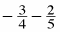2.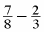3.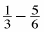4.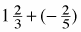5.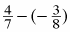6.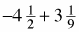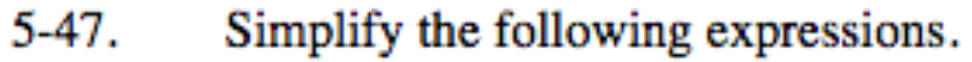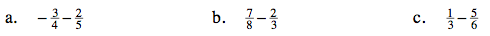Find a common denominator.

$-\frac{23}{20}$

Find a common denominator.

See part (b).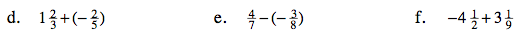Recall that adding a negative number is essentially subtraction.

Remember that when you subtract a negative number you are essentially adding it.

$\frac{53}{56}$

Remember, the solution will have the same sign as the larger number.

$-1\frac{7}{18}$